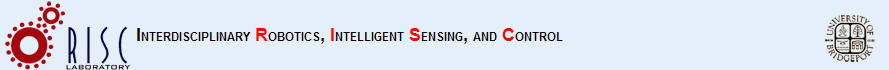# Autonomous quad player

Goal: To be able to have a quad copter autonomously hit a ball back.

This project consists of two main parts. Part one consists of being able to identify an object in a 3d space. Part two consits of having the quadcopter "know" where the object is and tap it. Part one is achieved by knowing where the object is at all times using two cameras to determine the x y z coordinates of such object. It is also important to know where the quadcopter is in conjuction with the object. This is done by capturing the frames that both cameras give us and extracting the location of each object on each frame.

The frames are captured using two Microsoft Kinects. These images are then converted utilizing Visual Studio C# language. The process is known as object extraction and it involves acquiring a certain amount of information that is then compared to a known value(in this case the color of the objects) and if found, a threshold size is compared and a location is then acquired. Each camera can give us the x and the y of the object. Since we need the z value as well , one camera serves as the x and the y while the other camera's x is the z value of the first camera.

Here is a demostration of the objects(balls) being added to the program and how it finds and gives us the location of the ball in the space provided. Notice that the slide values determine the final view as shown by the video. Also, there is some "tracking" being shown towards the end of the video.

For best results, change it to 480p and make it full screen